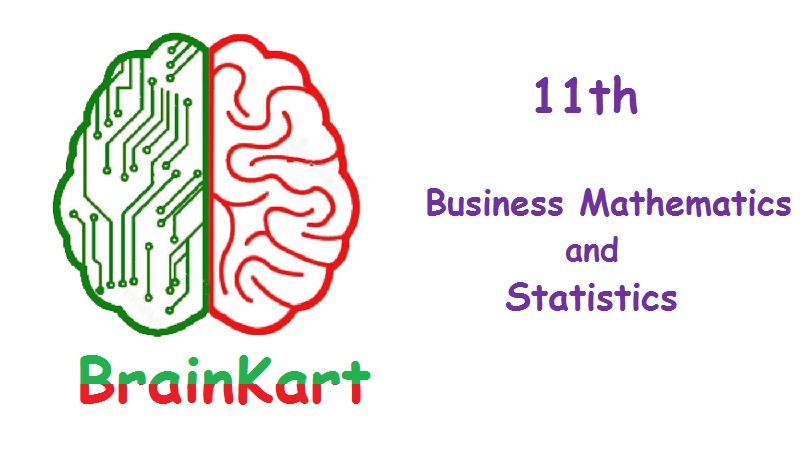## Online Study Material, Lecturing Notes, Assignment, Reference, Wiki and important questions and answers## Chapter 1 Matrices and Determinants

=> Determinants
=> Inverse of a matrix
=> Input - output Analysis

## Chapter 2 Algebra

=> Partial Fractions
=> Permutations
=> Combinations
=> Mathematical induction
=> Binomial theorem

## Chapter 3 Analytical Geometry

=> Analytical Geometry' Introduction
=> Locus
=> System of straight lines
=> Pair of straight lines
=> Circles
=> Conics

## Chapter 4 Trigonometry

=> Trigonometry: Introduction
=> Trigonometric ratios
=> Trigonometric ratios of compound angles
=> Transformation formulae
=> Inverse Trigonometric Functions

## Chapter 5 Differential Calculus

=> Differential Calculus: Introduction
=> Functions and their graphs
=> Limits and derivatives
=> Differentiation techniques